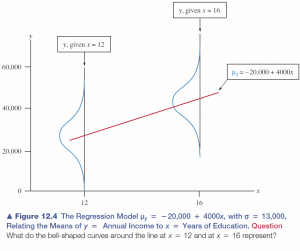8
Nov 16

## The pearls of AP Statistics 35

The disturbance term: To hide or not to hide? In an introductory Stats course, some part of the theory should be hidden. Where to draw the line is an interesting question. Here I discuss the ideas that look definitely bad to me.

### How disturbing is the disturbance term?

In the main text, Agresti and Franklin never mention the disturbance term$u_i$ in the regression model

(1)$y_i=a+bx_i+u_i$

(it is hidden in Exercise 12.105). Instead, they write the equation for the mean$\mu_y=a+bx$ that follows from (1) under the standard assumption$Eu_i=0$. This would be fine if the exposition stopped right there. However, one has to explain the random source of variability in$y_i$. On p. 583 the authors say: "The probability distribution of y values at a fixed value of x is a conditional distribution. At each value of x, there is a conditional distribution of y values. A regression model also describes these distributions. An additional parameter σ describes the standard deviation of each conditional distribution."

Further, Figure 12.4 illustrates distributions of errors at different points and asks: "What do the bell-shaped curves around the line at x = 12 and at x = 16 represent?"Figure 12.4. Illustration of error distributions

Besides, explanations of heteroscedasticity and of the residual sum of squares are impossible without explicitly referring to the disturbance term.

### Attributing a regression property to the correlation is not good

On p.589 I encountered a statement that puzzled me: "An important property of the correlation is that at any particular x value, the predicted value of y is relatively closer to its mean than x is to its mean. If an x value is a certain number of standard deviations from its mean, then the predicted y is r times that many standard deviations from its mean."

Firstly, this is a verbal interpretation of some formula, so why not give the formula itself? How good must be a student to guess what is behind the verbal formulation?

Secondly, as I stressed in this post, the correlation coefficient does not entail any prediction about the magnitude of a change in one variable caused by a change in another. The above statement about the predicted value of y must be a property of regression. Attributing a regression property to the correlation is not in the best interests of those who want to study Stats at a more advanced level.

Thirdly, I felt challenged to see something new in the area I thought I knew everything about. So here is the derivation. By definition, the fitted value is

(2)$\hat{y_i}=\hat{a}+\hat{b}x_i$

where the hats stand for estimators. The fitted line passes through the point$(\bar{x},\bar{y})$:

(3)$\bar{y}=\hat{a}+\hat{b}\bar{x}$

(this will be proved elsewhere). Subtracting (3) from (2) we get

(4)$\hat{y_i}-\bar{y}=\hat{b}(x_i-\bar{x})$

(using equation (4) from this post)$=\rho\frac{\sigma(y)}{\sigma(x)}(x_i-\bar{x}).$

It is helpful to rewrite (4) in a more symmetric form:

(5)$\frac{\hat{y_i}-\bar{y}}{\sigma(y)}=\rho\frac{x_i-\bar{x}}{\sigma(x)}.$

This is the equation we need. Suppose an x value is a certain number of standard deviations from its mean:$x_i-\bar{x}=k\sigma(x)$. Plug this into (5) to get$\hat{y_i}-\bar{y}=\rho k\sigma(y)$, that is, the predicted y is$\rho$ times that many standard deviations from its mean.